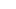top of page

# Math Tutoring

Math: Services## Start your path to success!

Math classes move too fast for many students, which leaves gaps in understanding and competency. Because math is always progressively building on itself, those little gaps can add up to large difficulties. We excel at finding those gaps in knowledge and patiently re-teaching them in a way that each student can understand. At the same time, we build understanding of current topics to avoid future gaps. We are familiar with APS standards (and shortcomings), and have tutored both CNM and UNM students.

We tutor the following math subjects:

Common Core

Middle School Math/Pre Algebra

Algebra 1 & 2

College Algebra

Linear Algebra

Pre Calculus

Calculus 1, 2, & 3

Geometry

Trigonometry

Statistics

Differential Equations

SAT/ACT Math

HESI MathThis channel is coming soon!
Math: Video Player
bottom of page